HOME plateWON | World!OfNumbers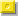Circular Primes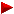Home PrimesPalindromic PrimesUndulating PrimesPalindromic Wing PrimesRepunits and their prime factors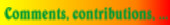Introduction

Circular Primes are primes with a special property.
One can find them by repeatedly chopping away the leftmost digit and appending them to the other end of the number.
Repeat this process until you come back at the starting number.
If all the intermediate formed numbers are prime then the starting number can be called 'circular'.
An example (e.g. with the number 1193) makes everthing clear

 1193 is prime 1931 is prime 9311 is prime 3119 is prime 1193 back to starting position

Circular Primes

It is immediate clear that a circular prime only consists of the digits 1, 3, 7 and 9.
The single-digit primes 2 and 5 are the only exceptions.
In the rightmost column of the table I put the total number of primes of the given length made of a mixture
of these four digits. Only these combinations are eligible to become a circular prime however small the chances may be.
An even digit arriving at the end of the number obviously makes the number composite.
Ending with 5 evidently makes the number divisible by 5.
Also mentioned, in case no circular primes were found, are the -near misses- meaning that only one combination failed.
It is possible that more near misses exists when the digits 2, 4, 5, 6 and 8 are allowed.
( Carlos Rivera examined this case more in detail : see Sloane's A045978 ).

Statistically speaking, it seems very unlikely that I will discover more of them as the length increases beyond 14.
Should one exist above length 14, the chances are very high that this number will become famous in numbertheory circles.
It might be a good idea to take Keith Devlin words into consideration.
I quote from his book All the Math that's Fit to Print, chapter 97 :

"For example, there are the so-called permutable primes. These are prime numbers that remain prime
when you rearrange their digits in any order you please. For example, 13 is a permutable prime, since
both 13 and 31 are prime. Again, 113 is a permutable prime, since it and each of the numbers 131 and
311 is prime. It is known that there are only seven such numbers within reasonable range (less than about
4 followed by 467 zeros, in fact). You now know two of them. Find the other five."

John Shonder (email) informed me on [ August 18, 1997 ] that the  repunit primes  are also circular primes by the definition
given above. Every single "permutation" of those repunital digits makes a prime, meaning that any arrangement of their digits
results in a prime. He admits that they are trivial or 'obvious' circular but they qualify just as well.
Indeed he's right and therefore I included them in the next table. Up to this moment only five repunit primes are known :
R2, R19, R23, R317 and R1031 (See Sloane's Encyclopedia of Integer Sequences).
The last one was discovered in 1986 by Williams and Dubner.
Furthermore these repunit primes are palindromic so there you have a connection with the rest of my website.
Hence I can talk about Circular Repunital Palindromic Primes.

 Justin Chan's (email)contribution[ July 11, 2008 ] I found this article at http://primes.utm.edu/ : Proves (actually re-summarizes) that a n-digit permutable prime (excluding repunits) does not exist for 3 < n < 6*10^175. In fact, the existence of large permutable primes is related to the distribution of primes of which 10 is a primitive root. ( Note by Toshio Yamaguchi for my readers [ January 23, 2011 ]circular primes differ from permutable primes !    The permutable primes are a subset of the circular primes.    Circular primes: 2, 3, 5, 7, 11, 13, 17, 37, 79, 113, 197, 199, 337, 1193, 3779, 11939, 19937, 193939, 199933    Permutable primes: 2, 3, 5, 7, 13, 17, 37, 79, 113, 199, 337 )

David W. Wilson (email) reports the following after letting me know there are no circular primes of length 10, 11 and 12:
"On statistical grounds, I would be extremely surprised if any longer circular primes exist. A cursory analysis:"

Using the estimate of n/log(n) primes <= n, we can estimate that there are about 10^d/log(10^d)
d-digit primes (this estimate is on the high side, since it includes primes with fewer than d digits).
The probability that a d-digit number is prime is therefore about
1/log(10^d).

A d-digit circular prime other than a repunit must generate d distinct values by cycling.
By the above estimate, the probability that these d values will all be prime is about
(1/log(10^d))^d,
p = 10^d/(log(10^d)^d  d-digit circular primes.

For various d, the values of p are:

```1   4.34294481903251827652
2   4.71529242529034823051
3   3.03381552720562897797
4   1.38962391600003339055
5   0.49439211408556231820
6   0.14381307270490575783
7   0.03538375334789643158
8   0.00754318214030964006
9   0.00141865311747126389
10  0.00023869488522460123```

This argument is obviously very imprecise, but I think the general conclusion is valid,
namely that the expected number of d-digit non-repunit circular primes rapidly
approaches 0 as d increases.

On [ August 13, 2000 ] Walter Schneider (email), the author who determinded there
are no new circular primes of lengths 17, 18 and 19,
kindly wrote some explanatory notes thus clarifying the methods and tools he used.

"I think my program to search for circular primes is very obvious.
The main steps for circular primes of length n are:

1. Recursively generate all possible strings of length n.

Only the digits 1, 3, 7 and 9 have to be considered. I use strings
(not numbers) because this is much faster. Because we search only for the
least number in each cycle the recursion can be speeded up by some easy
tests. For example, digits 2 to n cannot be smaller than the first digit.
(Therefore most of the running time is spent when the first digit is one!)

2. For each string generated in 1. of length n determine the whole cycle
and test if the original string is the least one in the cycle. Note that
we still work with strings not numbers.

3. For each string in the cycle convert the string to a big integer and
test for a small factor. I use the LiDIA bigint library and make a
divisibility test by all primes less than 100.

4. Not too much numbers are left at this step. For each number in the cycle
make a fermat test.

As you can see there is nothing very special about my search program. One of the crucial facts
I think is the fast generation using strings not numbers and the quick test in step 3."

Sources Revealed

Enormous amounts of research have been performed on repunits.
Here's a reflection of them in a few websites :Repunits Prime FactorsRepunit - E.W. WeissteinREP-UNIT & REP-DIGIT

 Neil Sloane's "Integer Sequences" Encyclopedia can be consulted online : Neil Sloane's Integer Sequences Entries about the subject are the following : %N Every permutation of digits is a prime. under A003459. %N (10^n - 1)/9 is prime. under A004023. %N Primes of form (10^n - 1)/9 (next terms are for n = 317, 1031). under A004022. %N Primes such that         (a) reversal of digits gives a prime,         (b) deleting any digit gives a prime, and         (c) reversing digits and deleting any digit gives a prime. under A055604. Click here to view some of the author's [P. De Geest] entries to the table. Click here to view some entries to the table about palindromes.

Circular prime from "The Prime Glossary".

Prime Curios! - site maintained by G. L. Honaker Jr. and Chris Caldwell
11 - The 1st prime repunit.
3304
193939
199933
285646799
(19 ones) 1111111111111111111 is a Repunit prime.
1111111111111111111
(23 ones) 11111111111111111111111 is a Repunit prime.
11111111111111111111111
317 - Number of digits in the 3rd prime repunit.
11111...11111 (317-digits)
The largest known Repunit prime has 1031 digits.
11111...11111 (1031-digits)

The Table

Circular Primes
Length 270343
Total = ?
R270343 New Probable Prime Repunit, R(270343)
Found by Maksym Voznyy [ July 11, 2007 ]
Length 109297
Total = ?
R109297 New Probable Prime Repunit, R(109297)
Found by Harvey Dubner [ March 28, 2007 ]
Length 86453
Total = ?
R86453 R86453 is a New Probable Prime Repunit
Found by Lew Baxter [ October 26, 2000 ]
Length 49081
Total = ?
R49081 New Probable prime Repunit, R(49081)
Found by Harvey Dubner [ September, 1999 ]
Length 1031
Total = ?
R1031 Repunit 1031
(10^1031-1)/9
Length 317
Total = ?
R317 Repunit 317
(10^317-1)/9
Length 25
Total = ?
Unknown. ?
Length 24
Total = ?
Unknown. ?
Length 23
Total = 1
R23 11111111111111111111111 is the only one !
Determined by W. Schneider & N. Pavlos
[ January 22, 2003 ]
Length 22
Total = 0
Nihil !
Determined by Walter Schneider
[ December 13, 2002 ]
Length 21
Total = 0
Nihil !
Determined by Walter Schneider
[ April 11, 2002 ]
Length 20
Total = 0
Nihil !
Determined by Walter Schneider
[ April 11, 2002 ]
Length 19
Total = 1
R19 1111111111111111111 is the only one !
Determined by Walter Schneider
[ August 10, 2000 ]
Length 18
Total = 0
Nihil !
Determined by Walter Schneider
[ July 28, 2000 ]
Length 17
Total = 0
Nihil !
Determined by Walter Schneider
[ July 25, 2000 ]
Length 16
Total = 0
Nihil ! Determined by Darren Smith
[ September 9, 1998 ]
Length 15
Total = 0
Nihil ! Determined by Darren Smith
[ August 19, 1998 ]
Length 14
Total = 0
Nihil ! Determined by Darren Smith
[ June 14, 1998 ]
Length 13
Total = 0
Nihil ! Determined by Darren Smith
[ June 12, 1998 ]
Length 12
Total = 0
Nihil !
Determined by David W. Wilson
[ February 18, 1998 ]
Length 11
Total = 0
Nihil !
Determined by David W. Wilson
[ February 17, 1998 ]
Length 10
Total = 0
Nihil !
Determined by David W. Wilson
[ February 17, 1998 ]
Length 9
Total = 0
Nihil !
```33191 eligible primes

One Near Miss exists

913311913 prime
133119139 prime
331191391 prime
311913913 prime
119139133 prime
191391331 prime
913913311 prime
139133119 prime
but 391331191 = 29 * 131 * 239 * 431   ```
Length 8
Total = 0
Nihil !
```9177 eligible primes

One Near Miss exists

71777393 prime
17773937 prime
77739371 prime
77393717 prime
73937177 prime
39371777 prime
93717773 prime
but 37177739 = 29 * 683 * 1877```
Length 7
Total = 0
Nihil !
```2709 eligible primes

Two Near Misses exist

a)   9197777 prime
1977779 prime
9777791 prime
7777919 prime
7779197 prime
7791977 prime
but 7919777 = 83 * 95419

b)   9991313 prime
9913139 prime
9131399 prime
1313999 prime
3139991 prime
1399913 prime
but 3999131 = 17 * 235243```
Length 6
Total = 2
193939
199933
```757 eligible primes
```
Length 5
Total = 2
11939
19937
```249 eligible primes
```
Length 4
Total = 2
1193
3779
```63 eligible primes
```
Length 3
Total = 4
113
197
199
337
```30 eligible primes
```
Length 2
Total = 5
11 = R2
13
17
37
79
```10 eligible primes
```
Length 1
Total = 5
(1)
2 *
3
5 *
7
```3 eligible primes
4 if 1 is taken as a prime

( apart from 2 and 5 )
```

Contributions

[ July 11, 2008 ]
Justin Chan (email) makes an important contribution ! - go to topic

[ April 11, 2002 ]
Walter Schneider (email) improved his search program forcircular primesconsiderably and used it to search for circular primes of
digit length 20 and 21. No solutions were found.
Running time on a Pentium-3 550Mhz was 19 hours for 20 digits
and a couple of days for 21 digits.

Walter Schneider (email) determined there are no circular primes for length 17.
The total running time on his Pentium-III 550 Mhz was 14 hours.
and three days later determined also none for length 18.
Total running time was 2 days.
Finally, [ August 10, 2000 ]  Walter determined that Repunit R19 is the only circular prime of length 19.

Darren Smith (email) from Milwaukee, Wisconsin determined there are no circular primes for lengths 13, 14, 15 and 16.

David W. Wilson (email) determined there are no circular primes for lengths 10, 11 and 12.

```

```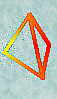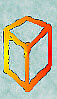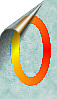[TOP OF PAGE]

Patrick De Geest - Belgium- Short Bio - Some Pictures